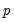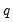arch
Estimate generalized autoregressive conditional heteroskedasticity (GARCH) models.
Syntax
eq_name.arch(p,q,options) y [x1 x2 x3] [@ p1 p2 [@ t1 t2]]
eq_name.arch(p,q,options) y=expression [@ p1 p2 [@ t1 t2]]
The first two options specify the order of the GARCH model:
The arch estimation method specifies a GARCH(p, q) model with p ARCH terms and q GARCH terms. Note the order of the arguments in which the ARCH and GARCH terms are entered.
The maximum value fororis 9; values above will be set to 9. The minimum value foris 1. The minimum value foris 0. If eitheroris not specified, EViews will assume a corresponding order of 1. Thus, a GARCH(1, 1) is assumed by default.
For Fractional Exponential GARCH models (FIEGARCH), EViews only estimates FIEGARCH(1,1) models. For these specifications,andoptions should not be specified, and if provided, will be ignored.
After the “ARCH” keyword and options, specify the dependent variable followed by a list of regressors in the mean equation.
By default, only the intercept is included in the conditional variance equation. If you wish to specify variance regressors, list them after the mean equation using an “@”-sign to separate the mean from the variance equation.
When estimating component ARCH models, you may specify exogenous variance regressors for both the permanent and transitory components. After the mean equation regressors, first list the regressors for the permanent component, followed by an “@”-sign, then the regressors for the transitory component. A constant term is always included as a permanent component regressor.
Options
Type Options
The default is to estimate a standard GARCH model. You may specify one of the followings keywords to estimate a different model:

 egarch Exponential GARCH. parch[=arg] Power ARCH. If the optional arg is provided, the power parameter will be set to that value, otherwise the power parameter will be estimated. cgarch Component (permanent and transitory) ARCH. figarch Fractional GARCH (FIGARCH). fiegarch Fractional Exponential GARCH (FIEGARCH(1,1)).
General Options

 thrsh=integer (default=0) Number of threshold terms for GARCH and Component models. The maximum number of terms allowed is 9. For Component models, “thrsh” must take a value of 0 or 1. vt Variance target of the constant term for GARCH models. (May not be used with integrated specifications.) integrated Restrict GARCH model to be integrated, i.e. IGARCH. (May not be used with variance targeting.) asy=integer (default=1) Number of asymmetric terms in Power ARCH or EGARCH models. The maximum number of terms allowed is 9. trunclag=integer (default=1000) Number of terms in the expansion approximation for FIGARCH and FIEGARCH models. archm=arg ARCH-M (ARCH in mean) specification with the conditional standard deviation (“archm=sd”), the conditional variance (“archm=var”), or the log of the conditional variance (“archm= log”) entered as a regressor in the mean equation. tdist [=number] Estimate the model assuming that the residuals follow a conditional Student’s t-distribution (the default is the conditional normal distribution). Providing the optional number greater than two will fix the degrees of freedom to that value. If the argument is not provided, the degrees of freedom will be estimated. ged [=number] Estimate the model assuming that the residuals follow a conditional GED (the default is the conditional normal distribution). Providing a positive value for the optional argument will fix the GED parameter. If the argument is not provided, the parameter will be estimated. z Turn of backcasting for both initial MA innovations and initial variances. backcast=n Backcast weight to calculate value used as the presample conditional variance. Weight needs to be greater than 0 and less than or equal to 1; the default value is 0.7. Note that a weight of 1 is equivalent to no backcasting, i.e. using the unconditional residual variance as the presample conditional variance. optmethod = arg Optimization method: “bfgs” (BFGS); “newton” (Newton-Raphson), “opg” or “bhhh” (OPG or BHHH), “legacy” (EViews legacy).“bfgs” is the default for new equations. optstep = arg Step method: “marquardt” (Marquardt - default); “dogleg” (Dogleg); “linesearch” (Line search).(Applicable when “optmethod=bfgs”, “optmethod=newton” or “optmethod=opg”.) b Use Berndt-Hall-Hall-Hausman (BHHH) as maximization algorithm. The default is Marquardt.(Applicable when “optmethod=legacy”.) cov=arg Covariance method: “ordinary” (default method based on inverse of the estimated information matrix), “huber” or “white” (Huber-White sandwich method), “bollerslev” (Bollerslev-Wooldridge method). covinfo = arg Information matrix method: “opg” (OPG); “hessian” (observed Hessian), “(Applicable when non-legacy “optmethod=” with “cov=ordinary”.) h Bollerslev-Wooldridge robust quasi-maximum likelihood (QML) covariance/standard errors. (Applicable for “optmethod=legacy” when estimating assuming normal errors.) m=integer Set maximum number of iterations. c=scalar Set convergence criterion. The criterion is based upon the maximum of the percentage changes in the scaled coefficients. s Use the current coefficient values in “C” as starting values (see also param). s=number Specify a number between zero and one to determine starting values as a fraction of preliminary LS estimates (out of range values are set to “s=1”). numericderiv / ‑numericderiv [Do / do not] use numeric derivatives only. If omitted, EViews will follow the global default. fastderiv / ‑fastderiv [Do / do not] use fast derivative computation. If omitted, EViews will follow the global default.Available only for legacy estimation (“optmeth=legacy”). showopts / ‑showopts [Do / do not] display the starting coefficient values and estimation options in the estimation output. coef=arg Specify the name of the coefficient vector (if specified by list); the default behavior is to use the “C” coefficient vector. prompt Force the dialog to appear from within a program. p Print estimation results.
Saved results
Most of the results saved for the ls estimation method are also available after ARCH estimation; see Equation::ls for details.
Examples
equation arc1.arch(4, 0, m=1000, cov=bollerslev) sp500 c
estimates an ARCH(4) model with a mean equation consisting of the series SP500 regressed on a constant. The procedure will perform up to 1000 iterations, and will report Bollerslev-Wooldridge robust QML standard errors upon completion.
The commands:
c = 0.1
equation arc1.arch(thrsh=1, s, mean=var) @pch(nys) c ar(1)
estimate a TARCH(1, 1)-in-mean specification with the mean equation relating the percent change of NYS to a constant, an AR term of order 1, and a conditional variance (GARCH) term. The first line sets the default coefficient vector to 0.1, and the “s” option uses these values as coefficient starting values.
The command:
equation arc1.arch(1, 2, asy=0, parch=1.5, ged=1.2) dlog(ibm)=c(1)+c(2)* dlog(sp500) @ r
estimates a symmetric Power ARCH(2, 1) (autoregressive GARCH of order 2, and moving average ARCH of order 1) model with GED errors. The power of model is fixed at 1.5 and the GED parameter is fixed at 1.2. The mean equation consists of the first log difference of IBM regressed on a constant and the first log difference of SP500. The conditional variance equation includes an exogenous regressor R.
Following estimation, we may save the estimated conditional variance as a series named GARCH1.
arc1.makegarch garch1
Cross-references
See “ARCH and GARCH Estimation” for a discussion of ARCH models. See also Equation::garch and Equation::makegarch.